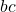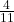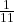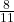How Cheenta works to ensure student success?
Explore the Back-Story

# Lines and Angles | PRMO 2019 | Question 7Try this beautiful problem from the PRMO, 2019 based on Lines and Angles.

## Lines and Angles - PRMO 2019

On a clock, there are two instants between 12 noon and 1 pm when the hour hand and the minute hand are at right angles. the difference in minutes between these two instants written as a+where a b c are positive integers withandin the reduced form. find a+b+c.

• is 107
• is 51
• is 840
• cannot be determined from the given information

### Key Concepts

Lines

Algebra

Angles

PRMO, 2019, Question 7

Higher Algebra by Hall and Knight

## Try with Hints

Minute hand turns 6 (in degrees) in 1 min and hour hand turns half (in degree) in 1 min

x min after 12 is 6x -=90 or 270 (in degrees) then x =16or 49Difference 32then a+b+c=32+8+11=51.

## Subscribe to Cheenta at Youtube

Try this beautiful problem from the PRMO, 2019 based on Lines and Angles.

## Lines and Angles - PRMO 2019

On a clock, there are two instants between 12 noon and 1 pm when the hour hand and the minute hand are at right angles. the difference in minutes between these two instants written as a+where a b c are positive integers withandin the reduced form. find a+b+c.

• is 107
• is 51
• is 840
• cannot be determined from the given information

### Key Concepts

Lines

Algebra

Angles

PRMO, 2019, Question 7

Higher Algebra by Hall and Knight

## Try with Hints

Minute hand turns 6 (in degrees) in 1 min and hour hand turns half (in degree) in 1 min

x min after 12 is 6x -=90 or 270 (in degrees) then x =16or 49Difference 32then a+b+c=32+8+11=51.

## Subscribe to Cheenta at Youtube

This site uses Akismet to reduce spam. Learn how your comment data is processed.

### Knowledge Partner